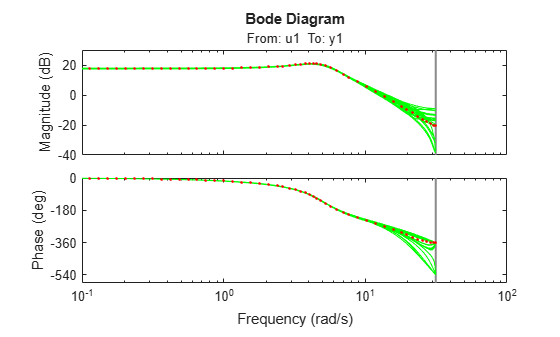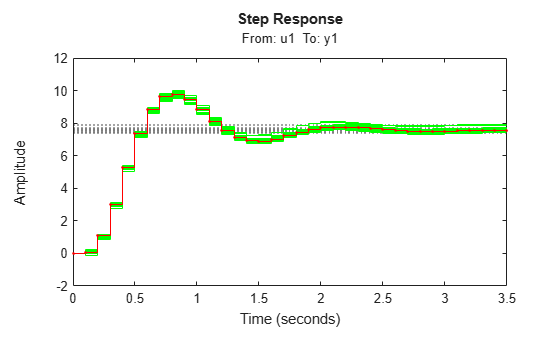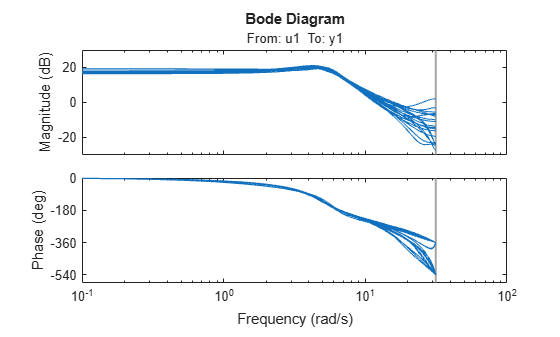# rsample

Random sampling of linear identified systems

## Syntax

``sysArray = rsample(sys,N)``
``sysArray = rsample(sys,N,stdDev)``

## Description

example

````sysArray = rsample(sys,N)` creates `N` random samples of the linear identified system `sys`. If you do not specify `N`, then `sysArray` contains ten random models.```

example

````sysArray = rsample(sys,N,stdDev)` specifies the standard deviation for randomly perturbing the parameters of `sys`.```

## Examples

collapse all

Estimate a third-order, discrete-time, state-space model.

```load iddata2 z2; sys = n4sid(z2,3);```

Randomly sample the estimated model.

```N = 20; sys_array = rsample(sys,N);```

Analyze the uncertainty in time (step) and frequency (Bode) responses.

```opt = bodeoptions; opt.PhaseMatching = 'on'; figure; bodeplot(sys_array,'g',sys,'r.',opt)``````figure; stepplot(sys_array,'g',sys,'r.-')```Estimate a third-order, discrete-time, state-space model.

```load iddata2 z2; sys = n4sid(z2,3);```

Randomly sample the estimated model. Specify the standard deviation level for perturbing the model parameters.

```N = 20; sd = 2; sys_array = rsample(sys,N,sd);```

Analyze the model uncertainty.

```figure; bode(sys_array);```Estimate an ARMAX model.

```load iddata1 z1 sys = armax(z1,[2 2 2 1]);```

Randomly sample the ARMAX model. Perturb the model parameters up to 2 standard deviations.

```N = 20; sd = 2; sys_array = rsample(sys,N,sd);```

Compare the frequency response confidence region corresponding to 2 standard deviations (asymptotic estimate) with the model array response.

```opt = bodeoptions; opt.PhaseMatching = 'on'; opt.ConfidenceRegionNumberSD = 2; bodeplot(sys_array,'g',sys,'r',opt)```To view the confidence region, right click the plot, and choose Characteristics > Confidence Region.

## Input Arguments

collapse all

Linear identified model, specified as one of the following model objects or an array of such objects.

Number of sample models to generate, specified as a positive integer.

Standard deviation for perturbing model parameters, specified as a positive scalar.

## Output Arguments

collapse all

Random models derived by sampling `sys`, returned as an array of identified models. These random models have the same structure as `sys`. Their parameter values are perturbed about the nominal values in a way that is consistent with the parameter covariance and based on the standard deviation `stdDev`.

If `sys` is a single model, then `sysArray` is an array of length `N` that contains randomized samples of `sys`.

If `sys` is an array of models, then the size of `sysArray` is equal to `[size(sys) N]`; that is, there are `N` randomized samples for each model in `sys`.

## Version History

Introduced in R2012a